## Air Humidity Tutorial (English)

Thomas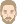Posts: 278
Joined: Sun Jun 19, 2005 10:33 pm -1100

### Air Humidity Tutorial (English)

Air Humidity Tutorial

The humidity data for the exterior or the interior air describe the moisture content of the air. In a hygrothermal simulation, the humidity of the ambient air is needed to determine
• the strength of the vapor diffusion exchange between the building component surface and the ambient air,
• the hygroscopic moisture content of a material exposed to the air.

There exists a variety of quantities describing the humidity of the air. Most of these humidity measures are in fact different physical quantities, not only different scales describing the same quantity (for example, degrees Fahrenheit and degrees Celsius are only two different scales describing the physical quantity "temperature"). While this variety may seem confusing, each of these quantities has its own advantages for certain applications. The user does not need to be familiar with these details to use the program, but the descriptions provided below may be helpful for a deeper understanding of hygric processes or for creating a user-defined weather file.

Humidity in WUFI

Different humidity measures are needed to describe different hygrothermal processes:

• In a hygrothermal simulation, vapor pressure differences are the driving force for vapor diffusion transport, both across the surfaces of a component and within the component. Diffusion processes are trying to equalize vapor pressure differences.

WUFI uses the humidity of the ambient air and the moisture content of the component surface to compute the vapor pressures on both sides of the surface - in the air and in the material. Their difference then determines the vapor diffusion flow across the surface. This vapor diffusion flow has to cross the boundary air layer which sticks to the surface and acts as a diffusion resistance. The diffusion flow across the surface is proportional to the driving vapor pressure difference and to the water vapor transfer coefficient which describes the properties of the boundary air layer.

Within the component, vapor pressure differences in the pore air also give rise to vapor diffusion transport, redistributing the moisture within the component. The diffusion resistance of the pore air is described by the μ-value of the material.

• If the surface temperature of the component falls below the dew point temperature of the ambient air, dew water deposition occurs.

• The equilibrium water content in a porous material exposed to ambient air is determined by the relative humidity of the air.

Moreover, differences of relative humidity within a porous material are the driving force for capillary transport of liquid water within the material. Capillary transport processes are trying to equalize differences in relative humidity. The intensity of the liquid flow is determined by the liquid transport coefficients.

If there is a temperature gradient across the building component, vapor pressure gradients and relative humidity gradients may point in opposite directions, giving simultaneously rise to vapor diffusion flows and liquid capillary flows which go in opposite directions. This is an example for a case where different humidity parameters are instrumental in determining the strengths and directions of different moisture transport mechanisms, possibly even acting simultaneously in opposite directions.

For a building component, this situation often occurs in winter when due to the low outdoor temperatures the indoor vapor pressure is higher than the outdoor vapor pressure while at the same time the outdoor relative humidity is higher than the indoor relative humidity.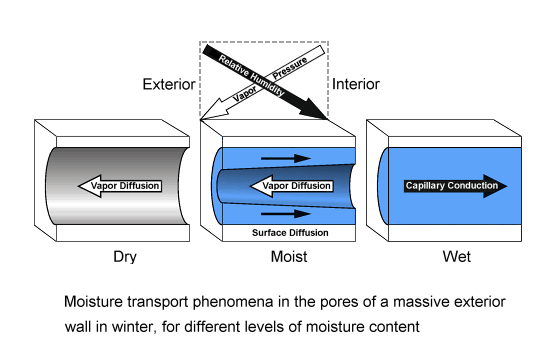Humidity Tutorial

Humidity Parameters

The climate file formats WUFI can read use the relative humidity as a measure of the moisture content of the air. However, if you are using weather data from other sources, you may encounter other humidity parameters. The following sections provide discussions of various popular humidity parameters for reference. Beyond the mere definitions, some background information about the parameters is presented which is not required to perform hygrothermal simulations but which may be helpful in understanding hygric processes.

Most of the humidity parameters depend not only on the amount of moisture in the air but also on temperature and/or pressure. That is, they change not only when water molecules are added to or removed from the air, but also when temperature or pressure changes occur. This is not a shortcoming of these parameters, it is appropriate for describing the respective properties of the moist air. However, you should be aware of these properties of the various humidity parameters to avoid misinterpretations.

In particular, the choice of an appropriate humidity parameter depends on whether only a quantitative statement about the amount of moisture in the air is intended, or whether one of the effects the moisture may have is to be described.

In hygrothermal building simulations where the pressure is usually the atmospheric pressure, the effect of pressure changes is usually negligible. It may be important in compressed air systems, for example.

• Water vapor partial pressure

The water vapor partial pressure, or water vapor pressure for short, is the pressure which the water vapor in the air contributes to the total pressure of the air (Dalton's law).

To a good approximation dry air and water vapor can be treated as ideal gases and do not affect each other in the mixture "moist air". Therefore, the ideal gas law applies to the water vapor contained in a given parcel of air: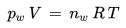pw [Pa] : Water vapor partial pressure in the parcel of air
V [m3] : Volume of the parcel of air
nw [mol] : mole number of the water vapor molecules in the parcel of air
R [J/(mol K)] : universal gas constant, 8.314 J/(mol K)
T [K] : absolute temperature of the parcel of air

Solving for the partial pressure pw gives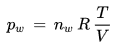As this equation shows, if the ratio T/V of the volume V and the temperature T of a given parcel of air is held fixed the water vapor pressure pw increases when water molecules are added to the parcel, because under these conditions the pressure of a gas is proportional to the number of its molecules (Avogadro's law). Under these conditions the water vapor pressure is a direct measure of the number of water molecules in the parcel.

In general, however, the water vapor pressure may also change without water molecules being added or removed. The water vapor pressure in a given parcel of air increases if the temperature T is raised at fixed volume V and fixed mole number nw (Charles's law, the molecules move faster), or if the volume V is compressed at fixed temperature T and fixed mole number nw (Boyle's law, the more concentrated molecules hit the container walls more often). The latter case is relevant for air compressors: If the air is compressed to such a degree that the increased vapor pressure of the contained water vapor exceeds the saturation vapor pressure at the current temperature, condensate is produced.

However, in hygrothermal simulations, usually none of the latter conditions applies. The air involved in a simulation (outdoor air, indoor air, pore air) is normally open to the ambient atmosphere, and it is therefore usually the total pressure which remains fixed in hygrothermal processes. Temperature is not fixed in such a simulation. The volume of a given parcel of air is not fixed either, it can freely contract and expand if its temperature or the total pressure change.

The ideal gas law also applies to the mixture "moist air", produced by mixing dry air and water vapor: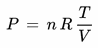P [Pa] : Total pressure of the parcel of air
(= sum of the partial pressures pdr of the dry air and pw of the water vapor in the parcel)
n [mol] : Total mole number of the parcel of air
(= sum of the mole numbers ndr of the dry air and nw of the water vapor in the parcel)

Dividing the ideal gas law for water vapor by the ideal gas law for moist air yields the following relationship between the water vapor partial pressure pw and the total pressure P:As the equation shows, the partial pressure of the water vapor can be found by multiplying the total pressure P of the mixture by the mole fraction nw/n of the water vapor. This is Dalton's Law.

The approximation made in the last step is justified for normal atmospheric air in which the mole number nw of the water vapor is small compared to the mole number ntr of the dry air (in saturated air at 20 °C there is one water molecule for every 43 air molecules). In this case - and for the mole number ntr of the dry air and the total pressure P held fixed - the partial pressure is a direct measure of the mole number of the water molecules in the air parcel.

The formula shows that the partial pressure can change - even with the number of water molecules held fixed - if total pressure varies. However, with the total pressure being prescribed, the partial pressure remains unaffected by changes of temperature or volume.

The meteorological variations of the total pressure only occur slowly and can probably be neglected in shorter data series. It may be necessary to take account of them when comparing different time periods or different locations.

The water vapor pressure can not exceed the saturation vapor pressure psat as long as there are surfaces nearby on which water can condense. Such surfaces are practically always present, for example wall surfaces (allowing dew deposition) or floating dust particles acting as condensation nuclei (allowing fog formation).
The saturation vapor pressure is strongly temperature-dependent. For example, at 0° C the saturation vapor pressure is 611 Pa, and at 20° C the saturation vapor pressure is 2339 Pa. A formula for calculating the saturation vapor pressure is given below.

Note: The limitation for the vapor pressure described above is often expressed by stating that at a given temperature the air can only hold a certain maximum amount of water vapor. This statement is misleading, however, since it implies that the maximum amount of water vapor is determined by the properties of the air - as if the air were a sponge which absorbs the moisture until it is filled to capacity. This is not the case - as mentioned above, there is virtually no interaction between the water vapor and the air. For example, in a pure noble gas atmosphere or even in a vacuum the maximum possible water vapor partial pressure is the same as in air. Furthermore, experience shows that other volatile liquids (such as gasoline or alcohol) can evaporate into "moisture-saturated air", so that the notion of an exhausted absorption capacity can not be correct.
Instead, the maximum possible partial pressure is determined by the properties of the water vapor itself, namely by the fact that condensability begins at the saturation vapor pressure. Short-term oversaturation can occur often, but due to condensation the partial pressure is then quickly reduced to saturation pressure (an example follows in the next section). In perfectly clean air with no nearby condensation surfaces (for example in the atmosphere at great height), saturation vapor pressure can be exceeded for prolonged time by a factor two or three.

Summary: Vapor pressure differences are the driving force for vapor diffusion, so they determine water vapor diffusion within the component and across the component surfaces. The amount of vapor in the air is limited by the saturation vapor pressure. Comparison of the current vapor pressure with the saturation vapor pressure corresponding to the current temperature allows to determine the possibility and rate of condensation.

• Relative Humidity

Relative humidity is defined as the ratio of the water vapor partial pressure present in the air and the water vapor saturation pressure corresponding to the current air temperature. It is expressed as a percentage (e.g. 78%) or as a fraction (e.g. 0.78).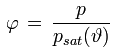φ [%] or [-] : relative humidity
p [Pa] : water vapor partial pressure
psat(ϑ) [Pa] : water vapor saturation pressure at temperature ϑ

Due to its definition, the dependence of the relative humidity on the number of water molecules in the air under given conditions is the same as the dependence of the water vapor partial pressure p under these conditions. In addition, however, there is a strong temperature dependence: If the temperature increases, the temperature-dependent saturation vapor pressure psat increases, and even in situations where the vapor pressure p remains constant, the relative humidity will decrease.

When the relative humidity of the air reaches 100 %, the air is saturated with humidity, and the partial vapor pressure is equal to the saturation vapor pressure. If further moisture is added, the moisture excess will condense out of the air (see above). Note that it is possible for a moist surface to give off moisture into the surrounding air even if that air is already saturated at 100 % relative humidity: If the temperature of the surface is higher than the temperature of the ambient air, the surface can have a higher partial vapor pressure, so that a diffusion flow from the surface into the air is created by the vapor pressure difference. This leads to oversaturation of the air close to the surface and moisture condensation in the air (which may be visible as little vapor plumes emanating from the surface).

The amount of equilibrium moisture adsorbed in the pores of a porous hygroscopic material depends on the ratio of the adsorption rate (depending on the water vapor pressure p in the pore air) and the evaporation rate (depending mainly on the saturation vapor pressure psat at the adsorbed water surfaces). It is therefore determined by the relative humidity φ of the pore air and, in consequence, by the relative humidity of the ambient air to which the material is exposed (rather than, say, its absolute humidity). The moisture storage function therefore describes the equilibrium moisture content as a function of relative humidity.
Most instruments for measuring the moisture content of the air use moisture-dependent properties of appropriate materials (e.g. change of length of fibres, change of electrical properties of polymers, etc). Since the moisture content of these materials is determined by the relative humidity of the air, the humidity parameter measured by these instruments is the relative humidity of the air.

The water vapor saturation pressure psat referred to in the above definition assumes chemically pure water and a flat water surface. If solutes (e.g. salts) are present in the water, or if the surface is concavely curved (e.g. in small pores), the actual saturation pressure is reduced and saturation equilibrium already occurs at less than 100% (since even under these circumstances pure water and a flat surface are used as reference by definition).

For example, the equilibrium vapor pressure over a saturated table salt solution at 20 °C is not 2339 Pa (the value over pure water). Due to the reduced concentration of water molecules in the solution it is only 1765 Pa, so that the air above the salt solution has an equilibrium humidity of 1765 Pa / 2339 Pa = 75.4 %. A saturated table salt solution can therefore be used to produce and maintain this relative humidity, one possible application being the conditioning of a material specimen in order to determine its moisture storage function for this humidity setting.

If the pore space of a porous material contains liquid water, the capillary forces cause the water surfaces in the pores to be concavely curved "menisci". Because the water molecules are bound more strongly in the curved surfaces, the equilibrium vapor pressure is reduced, as compared with the equilibrium pressure over a flat water surface.

The relative humidity of the pore air in hygric equilibrium with the curved menisci of the water surfaces is therefore less than 100 %, the exact value depending on the curvature of the menisci.
On the other hand, a curved meniscus exerts pulling forces on the water, creating a (negative) capillary pressure in the water, the exact value again depending on the curvature of the menisci.
In other words: In hygric equilibrium, there exists a mathematical relationship between the relative humidity in the pore air and the capillary pressure in the water. Since capillary pressure differences are driving the capillary liquid transport, it is equivalent to say that differences in relative humidity are driving liquid transport. In fact, WUFI's transport equations are using relative humidity as the driving potential because this has mathematical advantages over using the water content.

The Kelvin equation describes the functional relationship between the relative humidity of the pore air and the capillary pressure in the pore water: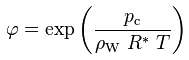φ [-] : relative humidity of pore air
pc [Pa] : capillary pressure in pore water, relative to the ambient pressure
ρW [kg/m3] : density of water, 1000 kg m-3
R* [J/(kg K)] : gas constant for water vapor, 461.5 J/(kg K)
T [K] : absolute temperature

The following table shows a few examples for the relationship between the curvature radius of the menisci, the capillary pressure created in the pore water by the curved menisci and the relative humidity of the pore air in hygric equlibrium with the curved menisci:The relationship between the curvature of the menisci (curvature radius r) and the capillary pressure pc is given by pc = 2 σ / r, where σ is the surface tension (0.072 N/m at 25°C). The relationship between the capillary pressure and the relative humidity is given by the Kelvin equation.

In a capillary with radius rc a meniscus will have the curvature radius r = rc / cos(γ). γ is the contact angle between the edge of the meniscus and the wall of the pore. For well-wettable materials, cos(γ) ≈ 1 and the meniscus radius in the table is approximately equal to the radius of the largest filled pores.

As an example from the table, the equilibrium humidity over partially filled pores with the radius 0.005 µm is about 80%. In other words: If the relative humidity of the pore air is 80%, the pores with radii less than 0.005 µm are filling up due to "capillary condensation" because in these pores 80% RH already correspond to oversaturation conditions. In small pores, liquid water can therefore begin to condense at relative humidities around 80..90% (depending on the pore radius distribution of the material under investigation), leading to a strong increase in absorbed water content for these moisture levels, a typical feature of the moisture storage functions of common materials.

• Water vapor concentration, absolute humidity

The water vapor concentration (or absolute humidity) is the ratio of the mass of the water vapor contained in a parcel of moist air to the volume of the parcel: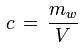c [kg/m3] : water vapor concentration, absolute humidity
mw [kg] : mass of water vapor contained in the parcel of air
V [m3] : volume of the parcel of air

The relationship between the water vapor concentration and the water vapor partial pressure is given by the ideal gas law: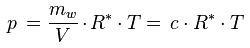p [Pa] : water vapor partial pressure
c [kg/m3] : water vapor concentration
R* [J/(kg K)] : gas constant for water vapor, 461.5 J/(kg K)
T [K] : absolute temperature

Solving for c gives: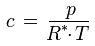As this formula shows, the dependence of the water vapor concentration on the number of water molecules in the air under given conditions is the same as the dependence of the water vapor partial pressure p under these conditions. However, there is an additional temperature dependence: If the temperature increases (at fixed total pressure, as is usually the case in hygrothermal simulations), the water vapor concentration in a given parcel of air decreases because then the air expands and the mass of water is spread over a larger volume V.

The water vapor concentration can be measured by using a strong absorbent to absorb all water contained in a known volume of air, and then weighing it to determine the mass of the absorbed water.

Typical values are 4.8 g/m3 at 0° C and saturation, and 17.3 g/m3 at 20°C and saturation.

• Mixing Ratio

The mixing ratio of a given parcel of moist air is the ratio of the mass of the water vapor to the mass of the dry air contained in the parcel: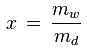x [kg/kg] : mixing ratio
mw [kg] : mass of water vapor in the parcel
md [kg] : mass of dry air in the parcel

The mixing ratio only changes when water molecules are added to or removed from the air. If the temperature of the parcel of air increases, its volume expands but the ratio between the number of water molecules and the number of air molecules in the parcel stays the same, and so does the ratio between their masses. The same is true for volume changes caused by pressure changes.

If, for example, moist air is pumped through pipes with different temperatures and pressures in different pipe sections, the mixing ratio of the air always stays the same along the way, as long as no evaporation and no condensation takes place.

The relationship between the mixing ratio and the water vapor partial pressure is given by the following formula: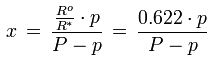x [kg/kg] : mixing ratio
Ro [J/(kg K)] : gas constant for dry air, 287.1 J/(kg K)
R* [J/(kg K)] : gas constant for water vapor, 461.5 J/(kg K)
p [Pa] : water vapor partial pressure
P [Pa] : barometric pressure

• Specific Humidity

The specific humidity of a given parcel of moist air is the ratio of the mass of the water vapor to the mass of the moist air (i.e. to the sum of the masses of the water vapor and the dry air) contained in the parcel: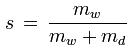s [kg/kg] : specific humidity
mw [kg] : mass of water vapor in the parcel
md [kg] : mass of dry air in the parcel

Just as with the mixing ratio, the specific humidity only changes when water molecules are added to or removed from the air. If the moist air consists almost entirely of water vapor and little air, the specific humidity approaches 1, while the mixing ratio approaches infinity.

The relationship between the specific humidity and the water vapor partial pressure is given by the following formula: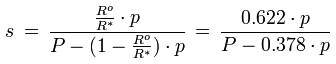s [kg/kg] : specific humidity
Ro [J/(kg K)] : gas constant for dry air, 287.1 J/(kg K)
R* [J/(kg K)] : gas constant for water vapor, 461.5 J/(kg K)
p [Pa] : water vapor partial pressure
P [Pa] : barometric pressure

• Dew Point Temperature

The dew point temperature of moist air is the temperature to which a parcel of moist air would have to be cooled (with the total pressure and the number of contained water molecules held fixed) for the contained water vapor to reach saturation and to begin condensation.

As mentioned above, the maximum water vapor pressure encountered at a given temperature ϑ is the saturation vapor pressure psat(ϑ).

The dew point temperature ϑdew of air containing water vapor with the vapor pressure p is the fictitious temperature for which one would have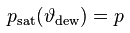psat [Pa] : saturation vapor pressure
ϑdew [°C] : dew point temperature
p [Pa] : current water vapor partial pressure

The dew point temperature therefore measures the water vapor pressure. It expresses the water vapor pressure in terms of the temperature which must be entered in a saturation vapor formula so that the formula returns the vapor pressure in question. Therefore, the remarks made above about the temperature and pressure dependence of the vapor pressure also apply to the dew point temperature.

The dewpoint temperature of air with a water vapor partial pressure of 611 Pa is 0 °C, the dewpoint temperature of air with a water vapor partial pressure of 2339 Pa is 20 °C.

The dew point temperature is a convenient humidity parameter to assess when condensation will occur.

The dew point temperature of the air can directly be measured with chilled-mirror hygrometers.

• Wet Bulb Temperature

The wet bulb temperature is the temperature indicated by a thermometer whose bulb is covered by a wet cloth sleeve. Due to evaporation cooling, this temperature is less than the air temperature indicated by a dry thermometer. The amount of cooling depends on the evaporation rate which is higher in dry air than in moist air. The conversion to other humidity parameters is done with psychrometer tables or empirical psychrometer formulas.

The wet bulb temperature of the air can directly be measured with suitably constructed thermometers.

Summary

The only commonly used humidity measures which only change when water molecules are added to or removed from the given parcel of air are the mixing ratio x and the specific humidity s. Therefore, these humidity measures describe the moisture content in the narrow sense.

The other humidity measures also change when pressure, volume or temperature change. For the intended statement this may be desirable or undesirable. The following table gives an overview of the additional variables affecting the various humidity measures (always assuming a constant number of molecules of dry air in the given parcel).

The table assumes that - as happens often in hygrothermics - the total pressure P is known or prescribed, so that the formulas are formulated using P wherever possible. If instead temperature and volume are known or prescribed, it may be more useful to reformulate the formulas accordingly, using the ideal gas law for the total pressure.
• The mixing ratio x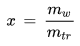is changed by
• adding or removing water molecules
.
• The specific humidity s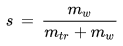is changed by
• adding or removing water molecules
.
• The water vapor partial pressure p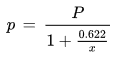is changed by
• adding or removing water molecules
• changing total pressure P
.
• The dewpoint temperature ϑdewis changed
• as the partial pressure p
.
• The water vapor concentration c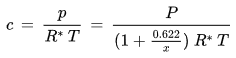is changed by
• adding or removing water molecules
• changing total pressure P
• changing temperature T
.
• The relative humidity φ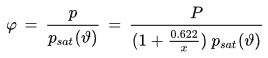is changed by
• adding or removing water molecules
• changing total pressure P
• changing temperature ϑ
For example, if a cold parcel of exterior air is brought into a warm room through an open window (assuming the same exterior and interior pressure P and no evaporation or condensation), then
• the mixing ratio x remains unchanged because no evaporation or condensation occurs
• the water vapor partial pressure p remains unchanged because the mixing ratio remains unchanged and the total pressure remains unchanged
• the water vapor concentration c decreases: While the water vapor partial pressure p remains constant, the temperature T increases.
• the relative humidity φ decreases: While the water vapor partial pressure p remains constant, the temperature ϑ and therefore the saturation pressure psat(ϑ) increase.
However, if the total pressure P of a parcel of air is reduced (for example, in an aircraft cabin while ascending, and no evaporation or condensation being assumed), then
• the mixing ratio x remains unchanged because no evaporation or condensation occurs
• the water vapor partial pressure p decreases proportional to the total pressure P
• the water vapor concentration c decreases proportional to the total pressure P (or to the water vapor partial pressure p). If the temperature changes, too, there is an additional temperature dependence.
• the relative humidity φ changes proportional to the total pressure P (or to the water vapor partial pressure p). If the temperature changes, too, there is an additional temperature dependence.
Saturation Vapor Pressure

The saturation vapor pressure is the equilibrium water vapor partial pressure prevailing over a flat water surface at a given temperature. There exists a large number of empirical saturation vapor pressure formulas, with different levels of complexity and accuracy. 'Magnus'-type formulas are popular because they are relatively simple and can explicitly be solved for the dew point temperature which corresponds to a given vapor pressure. An example for a Magnus-type formula and its inverse is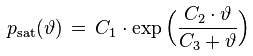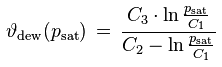psat [Pa] : saturation vapor pressure
ϑ [°C] : temperature
ϑdew [°C] : dew point temperature

with the following set of coefficients :Over a concavely curved water surface (e.g. a meniscus in a partially filled material pore), the saturation vapor pressure is less than over a flat surface. The very slight dependence of the saturation vapor pressure on the barometric pressure is usually ignored.

Literature:
 Deutscher Wetterdienst: Aspirations-Psychrometer-Tafeln.
5. Auflage, Vieweg

Update 2019-05-02, TS